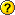#zhwiki

### Site Tools

wiki:mathjax_plugin

# MathJax Plugin

—- plugin —- description: Enables MathJax [http://mathjax.org] parsing of TeX math expressions in wiki pages author : Mark Liffiton email : [email protected] type : action, syntax lastupdate : 2021-11-20 compatible : Hogfather, Greebo, Frusterick Manners, Ponder Stibbons, Rincewind, Angua, Adora Belle, Weatherwax, Binky, 2014-09-29 “Hrun”, Detritus, Elenor of Tsort depends : conflicts : creole, indexmenu2, revealjs, s5 similar : jsmath, latex tags : math,tex,latex,mathjax

## Overview

This plugin adds MathJax to your wiki pages to let you easily write mathematical formulas that will be typeset and displayed cleanly. It is written to be as simple as possible; it loads and configures the script, protects TeX math expressions from other parsing, and no more.

It has been tested with MathJax version 2.7.x, the default MathJax version loaded by the plugin is 2.7.x, and all of the links to documentation here point to the v2.7 documentation. The plugin does not work with MathJax v3.x without modifications (work in progress).

## Installation

Search and install the plugin using the Extension Manager. Refer to Plugins on how to install plugins manually.

## Examples/Usage

$attr['_data']. "\n/*!]]>*/"; ### CDN Update - March, 2017 Until March 31, 2017, this plugin was using the MathJax CDN for loading MathJax. MathJax has shut down its CDN as of April 30, 2017. The default URL from which the plugin loads MathJax has been changed to use CDNJS as the MathJax maintainers recommend. If you have not changed the URL in your configuration, just update the plugin to its latest version, and your wiki should start using the new URL automatically. If you have modified the URL and are still using the MathJax CDN, you will need to update it in the wiki configuration manager (e.g. following the recommendation in the shutdown notice). And if you are not using the MathJax CDN, this should not affect you. ## FAQWhat happens if the Latex plug-in is installed simultaneously? Answer: I'm not certain (I don't have a place to easily install both), but if both are setup to use the same syntax for specifying math/equations, in the best case, the Latex plugin will capture/translate them and Mathjax won't see them. I wouldn't recommend trying it, though, as it will most likely just break things. Feel free to update this if you try it and find out what happens. Update: It seems to work fine. Assume settings for both plug-ins to be default. Result: 'Inline' Latex code like $a^2 + b^2 = c^2$ is processed by the Mathjax plugin. 'Display math mode' Latex code like \begin{equation} a^2 + b^2 = c^2 \end{equation} is processed (rendered as image) by the Latex plugin. Update: Recently, after fixing this bug, this code may be rendered by Mathjax as well. — Johan Tested with browsers: IE 8 and Firefox 13; PHP: QuickPHP 1.14.0; Dokuwiki: AnguaCan (large quantities of) equations be transferred from MS Word to the Wiki? Answer: Yes, using converters like: • Update: Do not expect too much from Mathtype. In Word 2007, simple symbols like the dot product or a hat (^) will abort the conversion to Latex. For automated batch conversion of even slightly complex math, you're screwed — JohanIs processing of Latex code disabled using syntax like %%$a^2 + b^2 = c^2$%% or <nowiki>$a^2 + b^2 = c^2$</nowiki>? Answer: No, Mathjax still renders the Latex code as if it is not wrapped by that syntax.How can I show the original Latex code without any formatting? Answer: Wrap the Latex code in code blocks, format it with the monospace style (e.g., ''$a^2$''), or escape dollar signs with backslashes (e.g., \$a^2\\$).What happens if the MathJax CDN server goes down?

Answer: Then Mathjax won't load, and the latex source code is shown instead of nicely rendered formulas.Are \newcommand and other custom macro/environment definitions supported?

Answer: Yes, either in your page inside math delimiters or through the configuration script.Will Mathjax work with PDF export plugins like dw2pdf?

Answer: Unfortunately, no. Mathjax renders all math formulas on the client-side (in your browser) using Javascript. The dw2pdf plugin creates PDFs on the server-side, where Javascript, and thus Mathjax, is unavailable. So any server-side export like that will contain the raw Latex code, not the rendered math formulas.

You can however export a PDF with the rendered math formulas from your browser by “printing to PDF.” This functionality is built in on OS X and Linux (look for “file” and “PDF” options in your print dialog), and you can add third-party “PDF printer” software to Windows.How to use (experimental) Extensions to MathJax (like siunitx.js)?

Answer: Get the script file, copy it to [home]/conf/ and then add the path to the file in the config manager (plugin»mathjax»configfile) like: conf/siunitx.jsHow to define global shortcuts / new commands?

Answer Add data/pages/mathjax.txt in the configuration manager under plugin»mathjax»configfile and then create the page mathjax with something simmilar to:

MathJax.Hub.Config({
TeX: {
Macros: {
RR: "{\\bf R}",
bold: ["{\\bf #1}",1],
Msun: "{\\textrm{M}_{\\odot}}"
}
}
});

(taken from the mathjax docu)How to configure the MathJax plugin step by step so that we can use XyJax in DokuWiki?

The section on third-party extensions that mentions XyJax has been updated to correct the URL for the new CDN. For instructions on installing/using XyJax, there's the XyJax README. It will involve modifying and hosting the XyJax javascript file yourself (it isn't available on a CDN as far as I can tell), which is outside of the scope of this plugin.

(Here are the steps of installation/configuration: a) Change the value of plugin»mathjax»url according to the Section of Third-Party Extensions. b) Follow the five steps of Installation Method 1 at the XyJax README. For details, see https://forum.dokuwiki.org/thread

1)
Accepted math environments (specified here in the code): align, align*, alignat, alignat*, displaymath, eqnarray, eqnarray*, equation, equation*, flalign, flalign*, gather, gather*, math, multline, multline*